# Gaussian Process Imputation/Forecast Models

By Matthew Barstead, Ph.D. | May 21, 2018

A well-established set of problems emerges when attempting to analyze non-stationary univariate time series (i.e., the signal’s mean and/or variance changes over time). A common approach is to impose some stationarity on the data so that certain modeling techniques can provide allow a research to make some predictions (e.g., ARIMA models). The selection of the appropriate assumptions to make when forcing a time series into stationarity is difficult to automate in many circumstances, requiring that a researcher evaluate competing models.

The models below will make use of the preloaded AirPassengers data in R. The data represent the total number of monthly international airline passengers (in thousands) from 1949 to 1960. It is easy to see these data have both a non-stationary mean and a non-stationary variance. There is also a clear periodic component to these data.

data('AirPassengers')
plot(AirPassengers)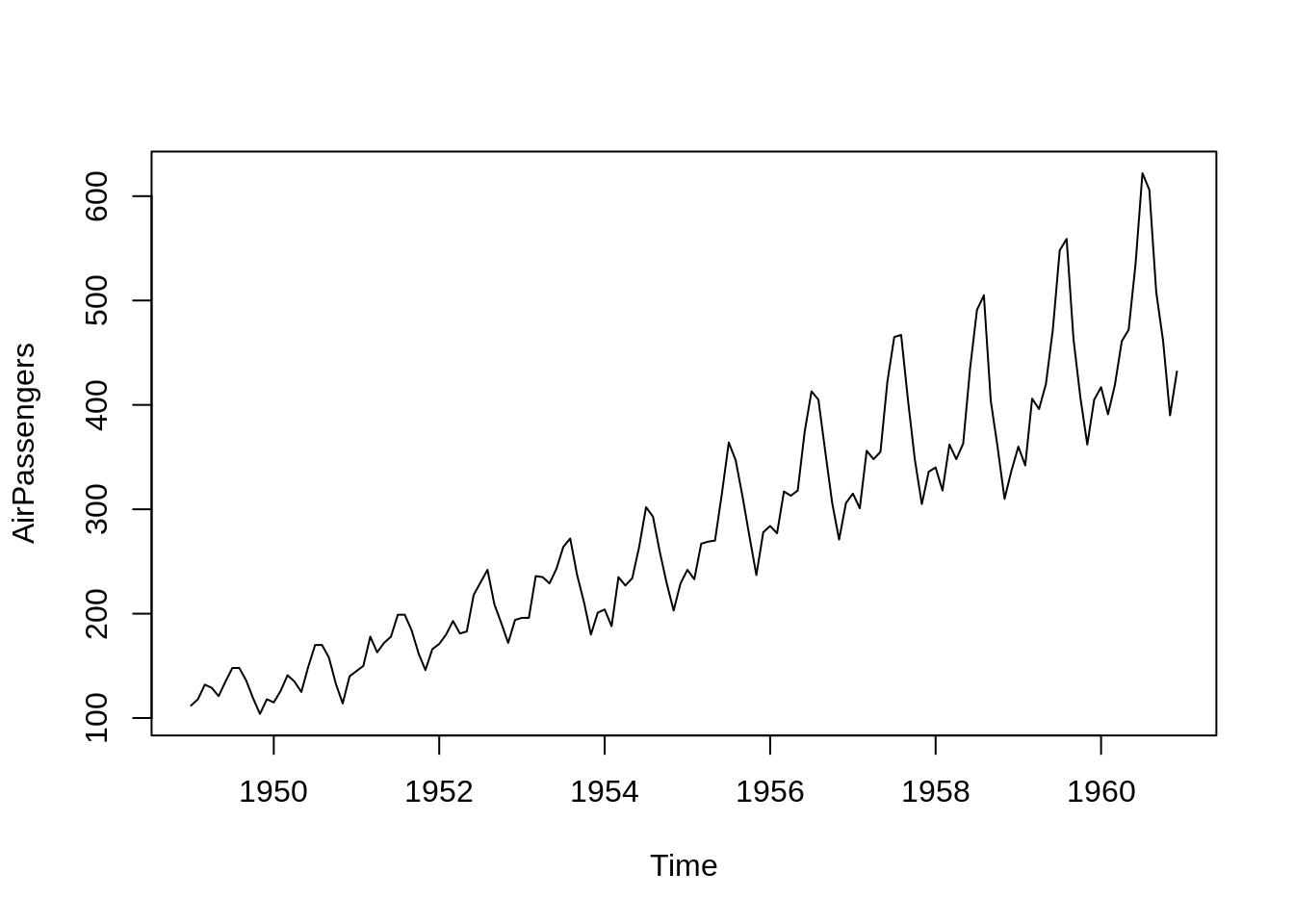As a toy problem, I am going to focus on the application of a Gaussian process model to forecasting future monthly passengers. This is not the only way one could try to solve this prediction problem. I offer it as a means of understanding the potential power that exists in using these sorts of models for prediction and imputation problems involving univariate time series data.

A few notes about Gaussian process models. To start they are a class of Bayesian models. There are a few R and Python packages that allow researchers to use this modeling approach. I have become something of a Stan convert recently, but it is not the only option out there.

The authoritative text on Gaussian process models was arguably published by Rasumssen & Williams in 2006, but only recently have computing power and programming languages allowed for a deeper tapping of this methodology’s strengths. For anyone interested in learning more about these models I highly recommend the Rasmussen & Williams (2006) text as a starting point.

It is worth pointing out that, because Guassian process models rely on Bayesian estimation, parameters either need to be fixed or given a prior distribution. I like that Bayesian analyses really make you think about your priors. It is the statistical equivalent of eating your veggies. You may not always enjoy it, but it will do you good in the long run. Strategies for choosing priors are beyond the purpose of this post. If interested, the following page on the Stan GitHub repo provides a brief, but reasonable overview as a starting point.

First, the data need a bit of prepping to be fed into a Stan program.

#Obtaining a numeric vector for time. Maintaining the units of measure at 1 = 1 year
Year<-seq(1949, 1960+11/12, by=1/12)

#converting time-series to a vector
Pass<-as.vector(AirPassengers)

#identifiying number of data points for the "training" data
N1<-length(Year)

#specifying 2-year prediction window.
year.fore2<-seq(1961, 1962+11/12, by=1/12)
N2<-length(year.fore2)

With the data prepped, I will run the first of two models. The first model relies solely on the squared exponential covariance function (plus error) to define the underlying Gaussian process. The squared exponential function takes the following form:

$k(t,t') = \sigma^2 exp\Big(-\frac{(t-t')^2}{2l_1^2}\Big)$

where $\sigma^2$ is the estimated variance accounted for by the function $k$ and $l$ is a length scale parameter that governs the decay rate. Smaller estimated values for $l$ indicate a faster decay rate in the covariance between two points as a function of time.

This model, along with its forecasting function are defined in Stan code below (Adapted from Nate Lemoine’s code):

functions{
//covariance function for main portion of the model
matrix main_GP(
int Nx,
vector x,
int Ny,
vector y,
real alpha1,
real rho1){
matrix[Nx, Ny] Sigma;

//specifying random Gaussian process that governs covariance matrix
for(i in 1:Nx){
for (j in 1:Ny){
Sigma[i,j] = alpha1*exp(-square(x[i]-y[j])/2/square(rho1));
}
}

return Sigma;
}
//function for posterior calculations
vector post_pred_rng(
real a1,
real r1,
real sn,
int No,
vector xo,
int Np,
vector xp,
vector yobs){
matrix[No,No] Ko;
matrix[Np,Np] Kp;
matrix[No,Np] Kop;
matrix[Np,No] Ko_inv_t;
vector[Np] mu_p;
matrix[Np,Np] Tau;
matrix[Np,Np] L2;
vector[Np] yp;

//--------------------------------------------------------------------
//Kernel Multiple GPs for observed data
Ko = main_GP(No, xo, No, xo, a1, r1);
for(n in 1:No) Ko[n,n] += sn;

//--------------------------------------------------------------------
//kernel for predicted data
Kp = main_GP(Np, xp, Np, xp,  a1, r1);
for(n in 1:Np) Kp[n,n] += sn;

//--------------------------------------------------------------------
//kernel for observed and predicted cross
Kop = main_GP(No, xo, Np, xp,  a1, r1);

//--------------------------------------------------------------------
//Algorithm 2.1 of Rassmussen and Williams...
Ko_inv_t = Kop'/Ko;
mu_p = Ko_inv_t*yobs;
Tau=Kp-Ko_inv_t*Kop;
L2 = cholesky_decompose(Tau);
yp = mu_p + L2*rep_vector(normal_rng(0,1), Np);
return yp;
}
}

data {
int<lower=1> N1;
int<lower=1> N2;
vector[N1] X;
vector[N1] Y;
vector[N2] Xp;
}

transformed data {
vector[N1] mu;
for(n in 1:N1) mu[n] = 0;
}

parameters {
real<lower=0> a1;
real<lower=0> r1;
real<lower=0> sigma_sq;
}

model{
matrix[N1,N1] Sigma;
matrix[N1,N1] L_S;

//using GP function from above
Sigma = main_GP(N1, X, N1, X,  a1, r1);
for(n in 1:N1) Sigma[n,n] += sigma_sq;

L_S = cholesky_decompose(Sigma);
Y ~ multi_normal_cholesky(mu, L_S);

//priors for parameters
a1 ~ student_t(3,0,1);
//incorporate minimum and maximum distances - use invgamma
r1 ~ student_t(3,0,1);
sigma_sq ~ student_t(3,0,1);
}

generated quantities {
vector[N2] Ypred = post_pred_rng(a1, r1, sigma_sq, N1, X, N2, Xp, Y);
}

The model takes just over a minute to run. For those of you who are computational gearheads, here is the hardware I am working with (with a total of 64GB of RAM):

benchmarkme::get_cpu()
## $vendor_id ##  "GenuineIntel" ## ##$model_name
##  "Intel(R) Core(TM) i7-8700K CPU @ 3.70GHz"
##
## $no_of_cores ##  12 A quick view of summary stats good convergence of estimates across the 6 chains. pars.to.monitor<-c('a1','r1','sigma_sq', 'Ypred') summary(fit.stan1, pars=pars.to.monitor[-4])$summary
##                  mean      se_mean           sd        2.5%          25%
## a1        2.064974195 2.117451e-02 1.2312158202 0.739681295  1.272435874
## r1       22.681133287 1.252456e-01 9.0005034579 9.102436133 16.045254783
## sigma_sq  0.003559372 5.819530e-06 0.0004251947 0.002818252  0.003254155
##                   50%          75%        97.5%    n_eff      Rhat
## a1        1.759559393  2.505894675  5.176821352 3380.974 1.0010353
## r1       21.367822936 28.012894270 42.692355288 5164.270 0.9996089
## sigma_sq  0.003524852  0.003823097  0.004468209 5338.262 1.0002208

… and traceplots demonstrate good mixing.

traceplot(fit.stan1, pars=c('a1', 'r1', 'sigma_sq'))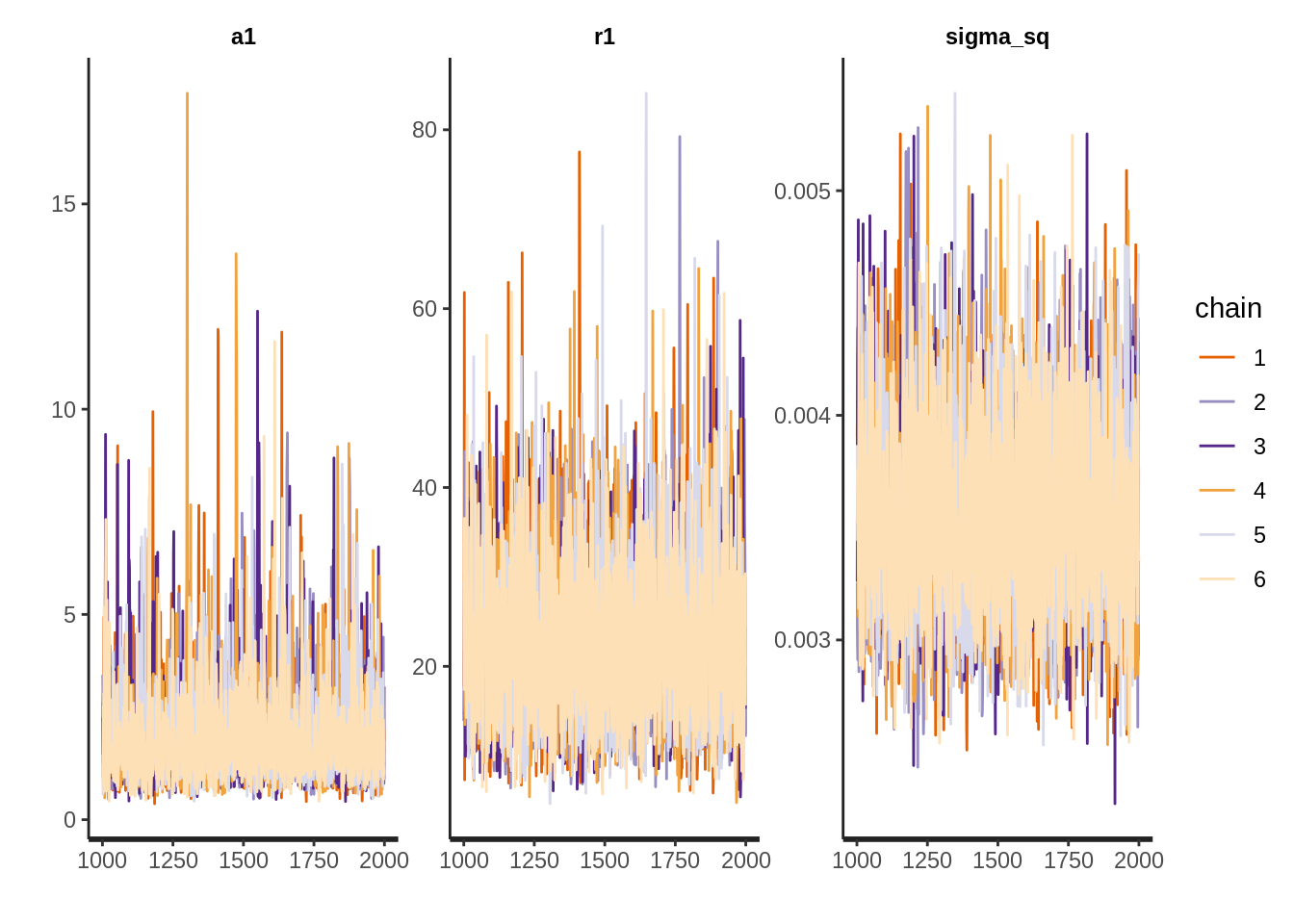So the real question is how did the model do?

Ypred<-10^colMeans(extract(fit.stan1, pars='Ypred')$Ypred) Ypred.DF<-extract(fit.stan1, pars='Ypred')$Ypred
UB<-vector()
LB<-vector()

for(i in 1:N2){
UB[i]<-10^quantile(Ypred.DF[,i], .975)
LB[i]<-10^quantile(Ypred.DF[,i], .025)
}

library(ggplot2)
DF.orig<-data.frame(Year=Year, Passengers=Pass)
DF.fore2<-data.frame(Year=year.fore2, Passengers=Ypred, UB=UB, LB=LB)

g1<-ggplot()+
geom_line(data=DF.orig, aes(x=Year, y=Passengers))+
geom_line(data=DF.fore2, aes(x=Year, y=Passengers))+
geom_ribbon(data=DF.fore2, aes(x=Year, ymin=LB, ymax=UB), alpha=.5)
g1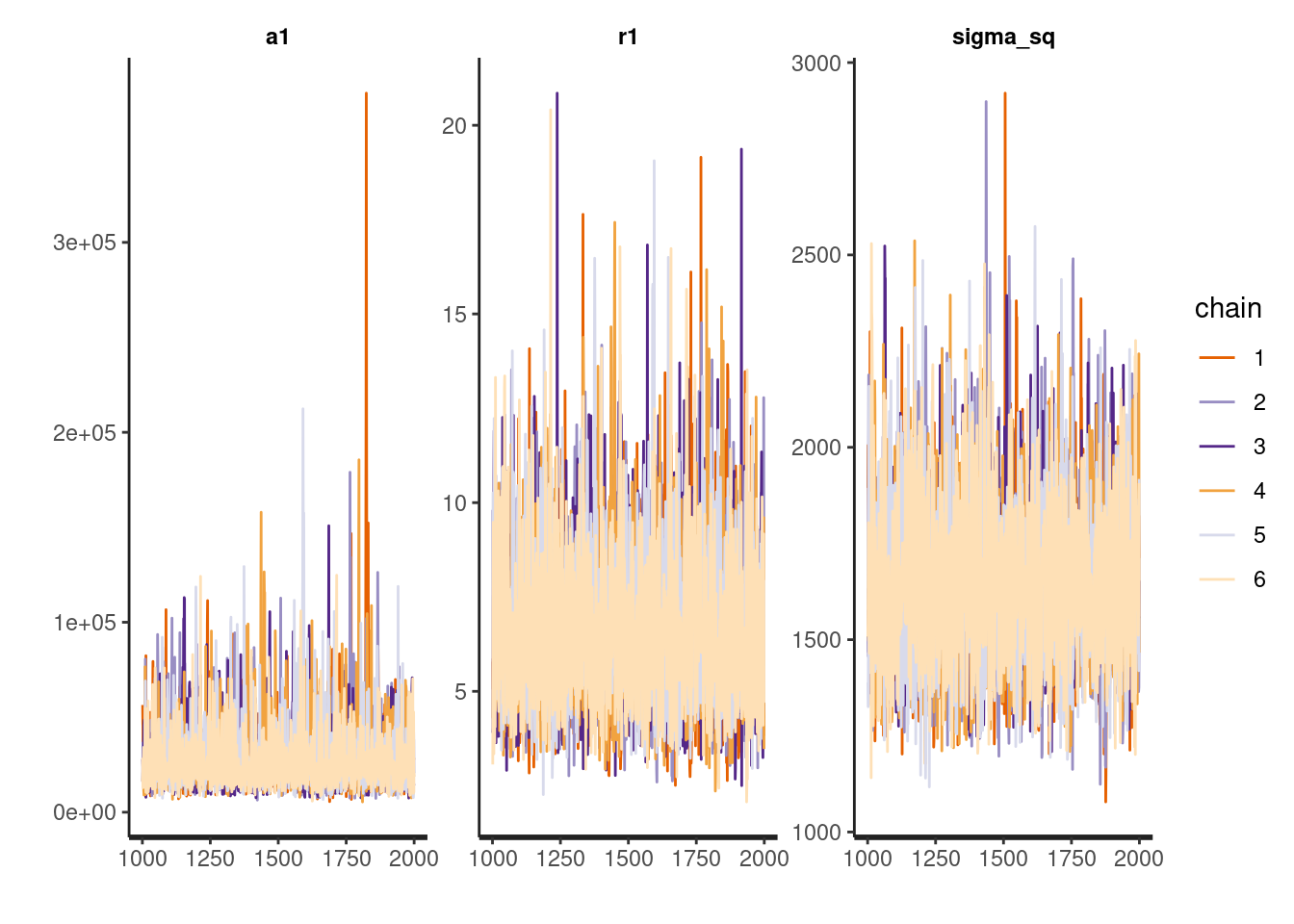Not all that great of a prediction to be honest. In this case, the function essentially reduces to a linear regression as there is no place for the periodic nature of the data to be explicitly modeled. This is where the flexibility of Gaussian process models starts to shine as any Gaussian process can be re-expressed as a the sum of an infinite number of Gaussian processes. Here we will add a covariance function that incorporates periodicity. In this case, a period is approximately one year.

The new periodic covariance function is:

$k(t,t')=\sigma_2^2 exp\Big(-\frac{2sin^2(\pi(t-t')*1)}{l_2^2}\Big) exp\Big(-\frac{(t-t')^2}{2l_3^2}\Big)$

The inclusion of the squared exponential function here simply reduces the ability of the annual features of the data to explain covariation as the interval between two points grows. Here is the Stan code.

functions{
//covariance function for main portion of the model
matrix main_GP(
int Nx,
vector x,
int Ny,
vector y,
real alpha1,
real alpha2,
real rho1,
real rho2,
real rho3){
matrix[Nx, Ny] K1;
matrix[Nx, Ny] K2;
matrix[Nx, Ny] Sigma;

//specifying random Gaussian process that governs covariance matrix
for(i in 1:Nx){
for (j in 1:Ny){
K1[i,j] = alpha1*exp(-square(x[i]-y[j])/2/square(rho1));
}
}

//specifying random Gaussian process incorporates heart rate
for(i in 1:Nx){
for(j in 1:Ny){
K2[i, j] = alpha2*exp(-2*square(sin(pi()*fabs(x[i]-y[j])*1))/square(rho2))*
exp(-square(x[i]-y[j])/2/square(rho3));
}
}

Sigma = K1+K2;
return Sigma;
}
//function for posterior calculations
vector post_pred_rng(
real a1,
real a2,
real r1,
real r2,
real r3,
real sn,
int No,
vector xo,
int Np,
vector xp,
vector yobs){
matrix[No,No] Ko;
matrix[Np,Np] Kp;
matrix[No,Np] Kop;
matrix[Np,No] Ko_inv_t;
vector[Np] mu_p;
matrix[Np,Np] Tau;
matrix[Np,Np] L2;
vector[Np] yp;

//--------------------------------------------------------------------
//Kernel Multiple GPs for observed data
Ko = main_GP(No, xo, No, xo, a1, a2, r1, r2, r3);
for(n in 1:No) Ko[n,n] += sn;

//--------------------------------------------------------------------
//kernel for predicted data
Kp = main_GP(Np, xp, Np, xp,  a1, a2, r1, r2,  r3);
for(n in 1:Np) Kp[n,n] += sn;

//--------------------------------------------------------------------
//kernel for observed and predicted cross
Kop = main_GP(No, xo, Np, xp,  a1, a2, r1, r2, r3);

//--------------------------------------------------------------------
//Algorithm 2.1 of Rassmussen and Williams...
Ko_inv_t = Kop'/Ko;
mu_p = Ko_inv_t*yobs;
Tau=Kp-Ko_inv_t*Kop;
L2 = cholesky_decompose(Tau);
yp = mu_p + L2*rep_vector(normal_rng(0,1), Np);
return yp;
}
}

data {
int<lower=1> N1;
int<lower=1> N2;
vector[N1] X;
vector[N1] Y;
vector[N2] Xp;
}

transformed data {
vector[N1] mu;
for(n in 1:N1) mu[n] = 0;
}

parameters {
real<lower=0> a1;
real<lower=0> a2;
real<lower=15> r1;      //Set after some preliminary modeling
real<lower=0> r2;
real<lower=0> r3;
real<lower=0> sigma_sq;
}

model{
matrix[N1,N1] Sigma;
matrix[N1,N1] L_S;

//using GP function from above
Sigma = main_GP(N1, X, N1, X, a1, a2, r1, r2, r3);
for(n in 1:N1) Sigma[n,n] += sigma_sq;

L_S = cholesky_decompose(Sigma);
Y ~ multi_normal_cholesky(mu, L_S);

//priors for parameters
a1 ~ normal(2.06,1.23);     //Taken from the first model
a2 ~ student_t(3,0,1);
//incorporate minimum and maximum distances - use invgamma
r1 ~ normal(22.68,9.005);   //Taken from the first model
r2 ~ student_t(3,0,1);
r3 ~ student_t(3,0,1);
sigma_sq ~ normal(0,1);
}

generated quantities {
vector[N2] Ypred = post_pred_rng(a1, a2, r1, r2, r3, sigma_sq, N1, X, N2, Xp, Y);
}


This model took about 6 minutes to run.

Unfortunately, the sampling algorithm that generates the Bayesian estimates is not parallelizable. Until Stan and rstan can run using graphics chips’ architecture (which has been buzzed about around the Stan ether), model run time is going to be the biggest downside. Still, 6 minutes is not that long to wait if the model performs well.

pars.to.monitor<-c(paste0('a', 1:2), paste0('r', 1:3), 'Ypred')
summary(fit.stan2, pars=pars.to.monitor[-6])$summary ## mean se_mean sd 2.5% 25% 50% ## a1 2.54983942 0.0134372272 0.92993236 1.03551274 1.842231419 2.464693706 ## a2 0.01028192 0.0007652084 0.02499935 0.00195483 0.003809465 0.005887629 ## r1 29.72788580 0.0860626598 5.77288905 19.05829543 25.751944510 29.510979184 ## r2 0.71435573 0.0024883370 0.12979765 0.49982165 0.627554970 0.700328139 ## r3 21.16693790 0.7029880494 10.71983939 2.13531511 14.836950073 19.220095144 ## 75% 97.5% n_eff Rhat ## a1 3.1470023 4.60109271 4789.4253 1.001067 ## a2 0.0100722 0.04229047 1067.3281 1.004514 ## r1 33.4589313 41.57756671 4499.4237 1.000575 ## r2 0.7850996 1.00378065 2720.9169 1.000556 ## r3 25.4484115 45.91201691 232.5309 1.026833 traceplot(fit.stan2, pars=pars.to.monitor[-6])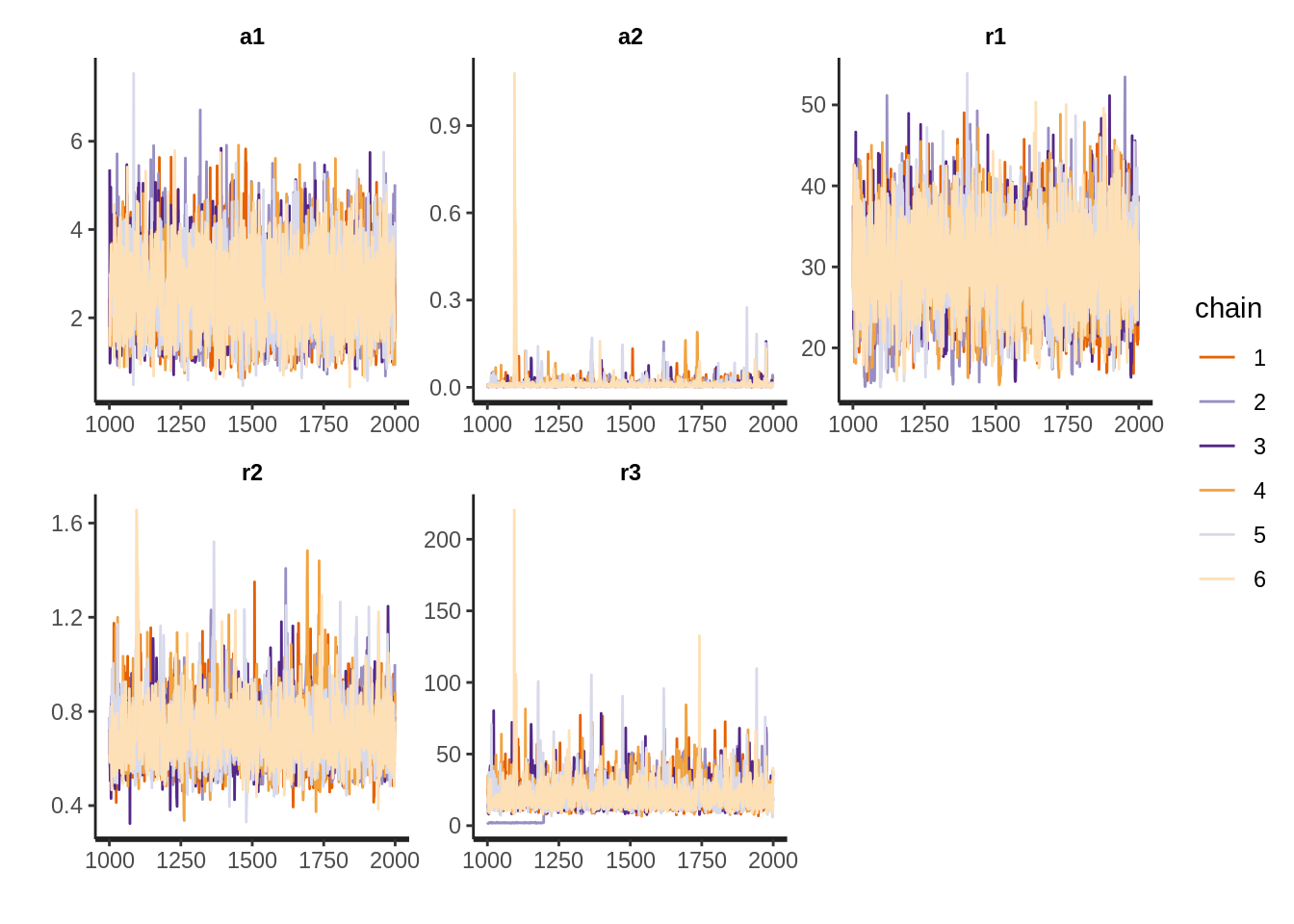Ypred<-10^colMeans(extract(fit.stan2, pars='Ypred')$Ypred)

Ypred.DF<-extract(fit.stan2, pars='Ypred')$Ypred UB<-vector() LB<-vector() for(i in 1:N2){ UB[i]<-10^quantile(Ypred.DF[,i], .975) LB[i]<-10^quantile(Ypred.DF[,i], .025) } library(ggplot2) DF.orig<-data.frame(Year=Year, Passengers=Pass) DF.fore2<-data.frame(Year=year.fore2, Passengers=Ypred, UB=UB, LB=LB) g1<-ggplot()+ geom_line(data=DF.orig, aes(x=Year, y=Passengers))+ geom_line(data=DF.fore2, aes(x=Year, y=Passengers))+ geom_ribbon(data=DF.fore2, aes(x=Year, ymin=LB, ymax=UB), alpha=.5) g1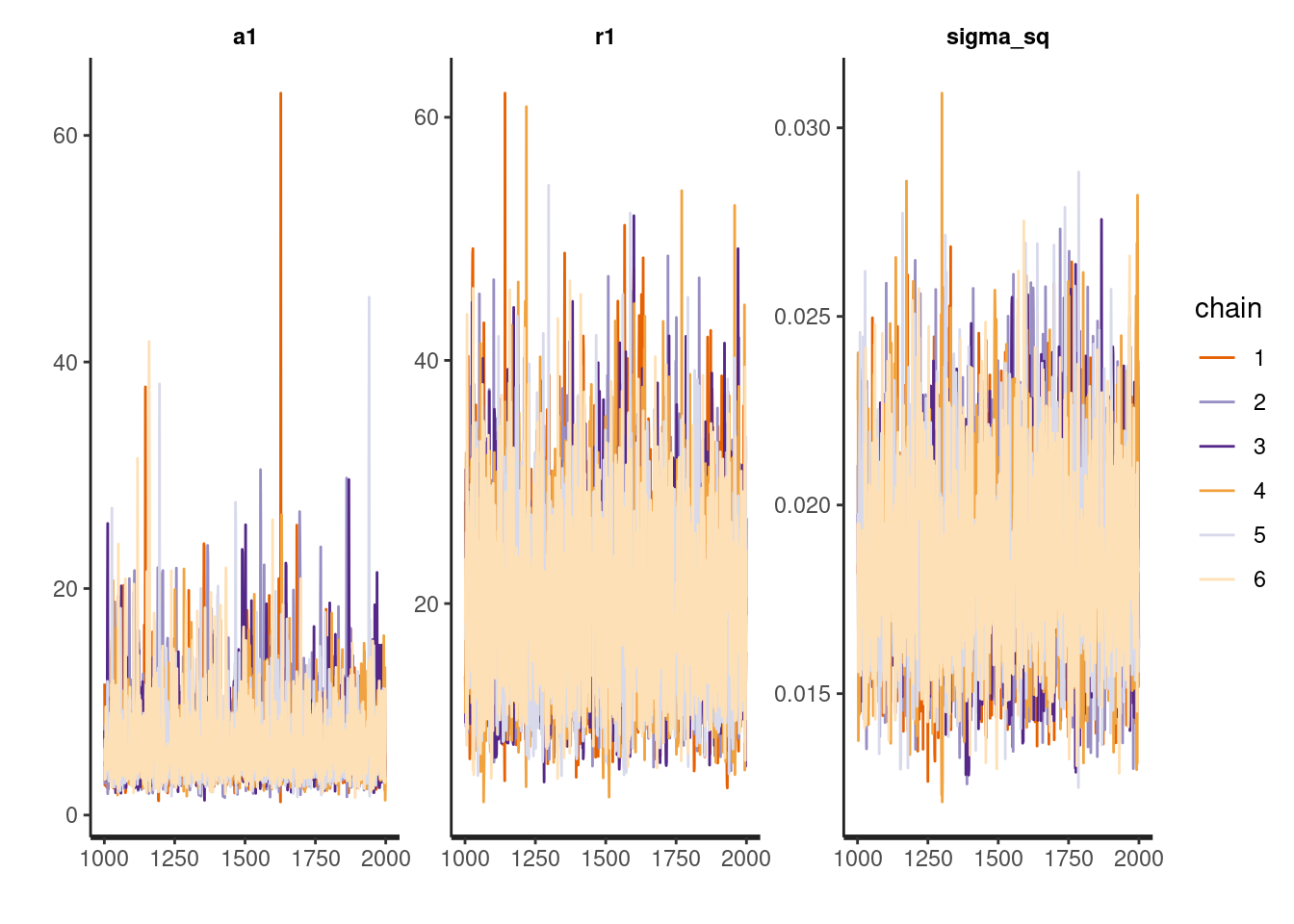The forecast looks to be in line with what I might expect based on trends leading up to 1962. The results are similar to those obtained using a different forecasting technique (i.e., an ARIMA model). (fit <- arima(log10(AirPassengers), c(0, 1, 1), seasonal = list(order = c(0, 1, 1), period = 12))) ## ## Call: ## arima(x = log10(AirPassengers), order = c(0, 1, 1), seasonal = list(order = c(0, ## 1, 1), period = 12)) ## ## Coefficients: ## ma1 sma1 ## -0.4018 -0.5569 ## s.e. 0.0896 0.0731 ## ## sigma^2 estimated as 0.0002543: log likelihood = 353.96, aic = -701.92 update(fit, method = "CSS") ## ## Call: ## arima(x = log10(AirPassengers), order = c(0, 1, 1), seasonal = list(order = c(0, ## 1, 1), period = 12), method = "CSS") ## ## Coefficients: ## ma1 sma1 ## -0.3772 -0.5724 ## s.e. 0.0883 0.0704 ## ## sigma^2 estimated as 0.0002619: part log likelihood = 354.32 pred <- predict(fit, n.ahead = 24) tl <- pred$pred - 1.96 * pred$se tu <- pred$pred + 1.96 * pred$se ARIMA.for<-data.frame(Year=year.fore2, Passengers=10^pred$pred, UB=10^as.numeric(tu), LB=10^as.numeric(tl))

g1<-ggplot()+
geom_line(data=DF.orig, aes(x=Year, y=Passengers))+
geom_ribbon(data=DF.fore2, aes(x=Year, ymin=LB, ymax=UB), alpha=.5, fill='blue')+
geom_ribbon(data=ARIMA.for, aes(x=Year, ymin=LB, ymax=UB), alpha=.5, fill='red')+
geom_line(data=DF.fore2, aes(x=Year, y=Passengers, color='blue'), lwd=1.25)+
geom_line(data=ARIMA.for, aes(x=Year, y=Passengers, color='red'), lwd=1.25)+
coord_cartesian(xlim=c(1960, 1963.25), ylim= c(275,1000))+
scale_color_manual(name='Forecast Model',
values = c('blue', 'red'),
labels = c('Gaussian Process', 'ARIMA'))
g1The two models make fairly similar predictions for 1961 (shaded regions represent respective 95% intervals). In 1962, the ARIMA model is a little more bullish than the Gaussian process model on airline passengers.

Still, it is impossible to know which of these models is better, a methodological question I may tackle in greater detail when I have some time. The answer is almost certainly “it depends.” For now, the main takeaway is that Gaussian process models may represent a useful approach to the age-old problems of forecasting and imputation, a fact I plan to exploit in some of my signal processing work.